# EMI Suppression Filters (EMC and Noise Suppression)Noise Suppression Products/EMI Suppression Filters

Noise Suppression Basic Course Section 1
Chapter 1
Chapter 2
Chapter 3
Chapter 4
Chapter 5
Chapter 6
Chapter 3

## Factors of making noise problems complex

### 3-1. Introduction

Chapter 2 has described the mechanism of causing electromagnetic noise and, among others, particularly described the noise that is generated from digital circuits in detail.
In order to handle the noise interference for electronic devices, you need to understand not only the noise source origin, but also the characteristics of the transmission lines and antennas. This chapter particularly describes the transmission lines among these.
So far, the noise occurrence has been explained with relatively simple expressions (except for harmonics). However, in order to explain the mechanism of noise transmission and emission, you need terms that are used for the transmission theory, electromagnetics and antenna theory as shown in Fig. 3-1-1. Without understanding these terms, you cannot handle the noise issues.
Therefore, this chapter will explain these terms (try to use the least number of formulas possible) as well as introducing important topics regarding noise such as resonance and damping, noise conduction and reflection, and source impedance.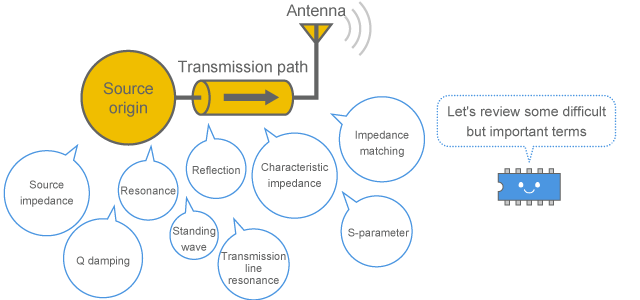Fig. 3-1-1 Contents to be explained in Chapter 3

### 3-2. Resonance and damping

Resonance is one of the important factors when causing noise or receiving a noise induction. If a circuit contains an unintended resonant circuit, it generates a very large current or voltage at the resonance frequency making it more likely to cause noise interference. It is important to eliminate resonance from the circuit as much as possible. In order to suppress resonance, damping resistors are used. This section will describe resonance and damping resistors.

#### 3-2-1. Parallel resonance and series resonance

##### (1) LC resonant circuit

Resonance means that the inductive reactance and capacitive reactance in a circuit cancel with each other at a certain frequency, and the specific frequency is called "resonance frequency." Although the typical parts that can generate a reactance (imaginary component of impedance) include inductors (coils) and capacitors, any other parts (even a simple wire) can be an element of resonance as those still contain a very small reactance. (Although a resonance in association with EMC can be caused by antenna, parallel plates and transmission line, etc. apart from the above, we only focus on LC resonance by inductor and capacitor).

##### (2) Impedance of resonant circuit

As shown in Fig. 3-2-1, there are two types of resonant circuit: series resonance and parallel resonance. As shown in the calculation example of Fig. 3-2-2, a series resonance makes the impedance minimum (ideally zero), while a parallel resonance makes it maximum (ideally ).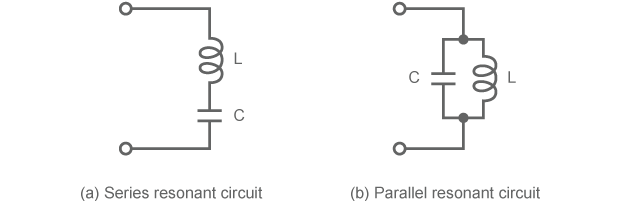Fig. 3-2-1 Series resonance and parallel resonance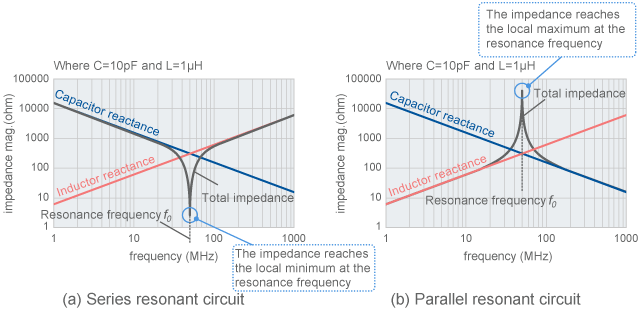(The graphs show the magnitude of reactance on a logarithmic axis)

Fig. 3-2-2 Impedance of resonant circuit

##### (3) Reactance is cancelled to zero

As shown in Fig. 3-2-3, it is understood that the magnitudes of the inductor reactance and capacitor reactance become the same at the resonance frequency, cancelling with each other and thus making it the total of zero.
Although Fig. 3-2-3 explains the case of series resonance, the reactance is replaced with susceptance (imaginary component of admittance) in the case of parallel resonance, resulting in cancelling the susceptance to zero at the resonance frequency. Therefore, it is easily understood that the impedance becomes maximum.

##### (4) Resonance frequency

The resonance frequency ƒ 0 can be estimated by the formula below for both series resonance and parallel resonance. In the example of Fig. 3-2-2, ƒ 0 is approx. 50MHz.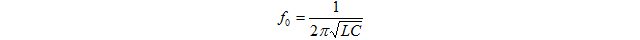(Formula 3-2-1)
##### (5) Resonance Q

The strength of this resonance is expressed by an index called Q (Quality factor). A higher Q indicates a stronger resonance. The index Q is also used as an index to represent the performance of capacitor and inductor. There is a relationship that when you use a capacitor or inductor with a large Q, you can create a resonant circuit with a large Q. How to estimate Q will be explained in Section 3-2-5.

##### (6) Self-resonance of capacitor and inductor

When a capacitor or inductor is used in the high frequency range, the part itself causes a resonance at a certain frequency due to the parasitic components inherent in the part. This is called self-resonance. Self-resonance will be further described in Chapter 6.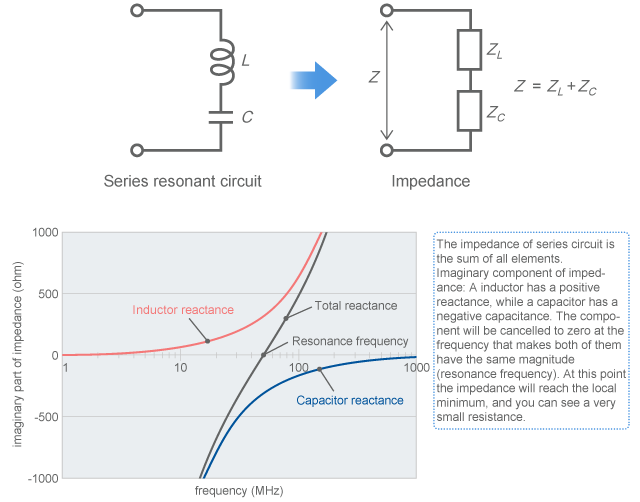Fig. 3-2-3 Mechanism of making the impedance minimum due to series resonance

#### 3-2-2. Issues of EMC measures for resonant circuit

##### (1) Resonant circuit amplifies the voltage

If there is an unintended resonance in the electric circuit, the impedance significantly changes at the resonance frequency resulting in a large current or voltage, which can be a cause of noise interference.
Let's say, for example, an alternating input signal is applied from the outside to the series resonant circuit that was calculated in Fig. 3-2-2(a). As shown in Fig. 3-2-4, when a signal generator with an output impedance of 50 ohms is used to apply a signal with a constant voltage (magnitude 0.5V), a voltage that is several times larger than the input signal occurs at the capacitor at the resonance frequency of 50MHz. In this case, the voltage occurred at the capacitor or inductor reaches to the input voltage multiplied by Q. How to estimate Q will be explained in Section 3-2-5. The conditions of Fig. 3-2-4 indicates Q = 6.3.

##### (2) Resonant circuit can be created unintentionally

The test circuit in Fig. 3-2-4 comprises a capacitor and inductor, where the constants used are values that normally occur in digital circuits. For example, the input terminal of an digital IC has a floating electrostatic capacitance of several pF. And the wiring has an inductance of about 1uH per meter. Therefore, if a cable of about 1m is connected to the input terminal of a digital IC (e.g. for connecting it to an external sensor), a resonant circuit like the one shown here will be created.

If a conductor is carelessly connected to this point, it can be a cause of noise emission.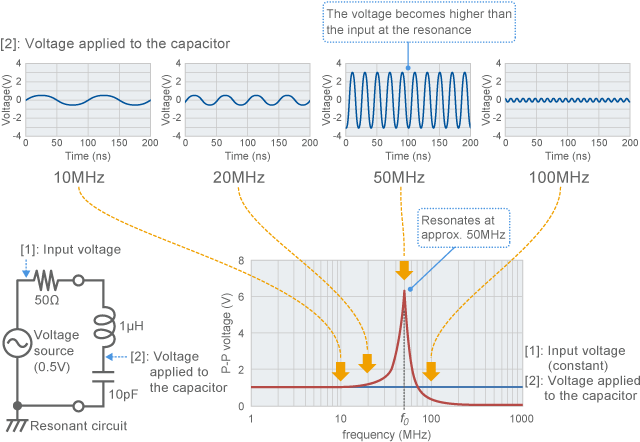Fig. 3-2-4 Example of frequency characteristics of resonant circuit (calculated value)

##### (3) The internal voltage becomes high even though the input voltage is only small

As shown in Fig. 3-2-2(a), the impedance of series resonant circuit becomes minimum at the resonance frequency. Thus, you might simply assume that the voltage becomes smaller. Why does the voltage actually become higher?
Fig. 3-2-5 shows the breakdown of the voltage. The voltage at the entrance (middle point between resistor and inductor) of the resonant circuit is surly reduced to a very small voltage. However, the current has been increased due to the reduced impedance. Therefore, a voltage larger than the applied voltage occurs inside the resonant circuit.
Why the voltage at the entrance of the resonant circuit disappears even though the capacitor receives some voltage? At this moment, the inductor also receives exactly the same voltage as that of the capacitor. Since the direction of this voltage is opposite to the capacitor, you can hardly see any voltage at the entrance of the resonant circuit.

##### (4) Resonant circuit has a completely different voltage depending on the point

When a circuit is resonating, the voltage appears to be significantly different depending on the point. Even if the voltage measurement at a point seems to indicate that noise has been reduced, the measurement of the total noise emission could be unchanged or even increasing. So care needs to be taken.
The above example is about the case of series resonant circuit. In the case of parallel resonant circuit, the current flowing through the capacitor and inductor becomes greater than the input signal. Since this current can also be a cause of noise, care needs to be taken in the case of parallel resonant circuit too.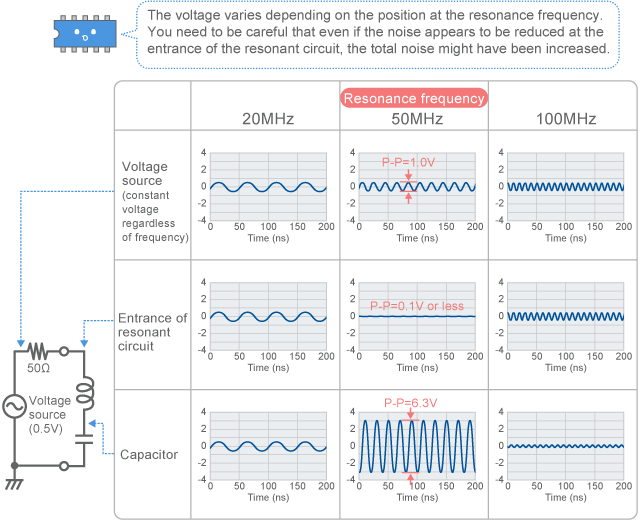Fig. 3-2-5 Voltage of different parts of resonant circuit (calculated value)

#### 3-2-3. When a digital circuit is connected to a resonant circuit

##### (1) Noise is more likely to occur at the resonance frequency

As described above, if a conductor that can work as an antenna has been connected to a resonant circuit, it picks up the high voltage of the resonance frequency and generates a strong emission, thus causing noise. In addition, in terms of immunity, noise can be easily received at the resonance frequency.
If such a resonant circuit with an attached antenna is connected to a signal that contains a wide range of frequencies such as a digital signal, the harmonics at frequencies that are close to the resonance frequency will have a strong emission. Fig. 3-2-6 and Fig. 3-2-7 show examples of the measurement of changes in pulse waveform and emission when the 50MHz series resonant circuit explained above is connected to a 10MHz clock signal. As an example of noise suppression, the waveform and emission with a ferrite bead attached are also shown here.

##### (2) When a digital signal is connected to a resonant circuit

Fig. 3-2-6 shows the test circuit and the measurement results of the voltage waveform. 74AC00 has been used for the digital ID that works as a noise source origin. The output of this IC is connected to the series resonant circuit with the resonance frequency of 50MHz. You can see that the observed waveform indicates a strong ringing in the 10MHz digital pulse, making the pulse waveform seriously distorted. This is considered to be because only the 5th order harmonic (50MHz) has been extracted among the harmonics contained in the 10MHz signal. (A ringing frequency of 50MHz has been observed)

##### (3) Damping with use of ferrite beads

As described later, damping resistors and ferrite beads are effective to suppress this type of resonance. Fig. 3-2-6 shows a waveform when a ferrite bead has been attached. You can see that the resonance has been suppressed and the signal has been brought back to the original pulse waveform.

##### (4) Resonance is confirmed by noise emission

Fig. 3-2-7 shows the results of noise emission. The electric field strength has been measured by the 3 meter method. For your reference, the figure also shows the measurement result without an antenna, and it has been confirmed that there is almost no noise emission when it only consists of a digital IC and resonant circuit as above. The line in the lower part of the figure indicates the dark noise level of the spectrum analyzer.

##### (5) LC resonance and antenna resonance

Fig. 3-2-7(a) shows the case that a resonant circuit is connected with a 15cm wire as an antenna to emit noise. A strong emission has been observed at the resonance frequency of 50MHz for the LC resonant circuit. Apart from the frequency, noise has also been observed at 500MHz. At this frequency, the 15cm wire attached as an antenna works as a 1/4 wavelength antenna. Therefore, you can possibly observe the resonant effect of the antenna in addition to the LC resonance in Fig. 3-2-7(a). The resonance of antenna will be described in a later section.
Fig. 3-2-7(c) shows a measurement result with a ferrite bead attached. You can see that the noise emission has been effectively suppressed.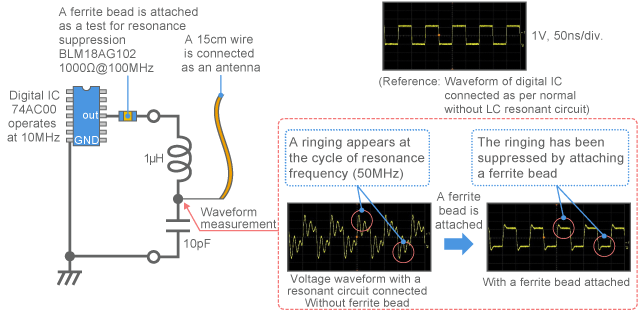Fig. 3-2-6 Test circuit with a resonant circuit and antenna connected to a digital signal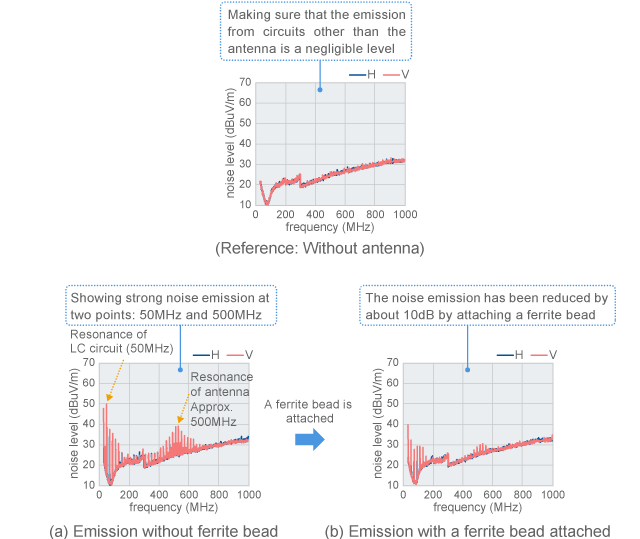Fig. 3-2-7 Noise emission with a resonant circuit and antenna connected to a digital signal

#### 3-2-4. Example of causing resonance without any inductor or capacitor

##### (1) Resonant circuit created by digital signal line

In order to take a measurement with an emphasis on the effect of resonance in Figs. 3-2-6 and 3-2-7, the experiment was carried out by creating a LC resonant circuit with a capacitor and inductor. However, resonance can occur in an actual circuit without such parts.
For example, in the case of digital signal wiring as shown in Fig. 3-2-8, the wire that connects between the driver and receiver has an inductance. In addition, the input terminal of the receiver that receives the signal has an electrostatic capacity. It is considered that the digital circuit described in Section 2-4-7 forms a resonant circuit with these factors.

##### (2) Problem becomes apparent as the resonance frequency becomes lower

When the digital signal wiring is very short, the resonance frequency by these factors becomes very high (several 100MHz) and thus the effects are negligible. However, if using a double-sided board, or if the inductance is increased by extending the wiring, or if the electrostatic capacity is increased by connecting multiple receivers, the effects (distortion to pulse waveform or increased noise emission etc.) from the lowered resonance frequency will no longer be negligible.
In preparation for the above cases, the signal output part can be provided with lands to allow using a resonance suppression component such as a ferrite bead so that the noise suppression measures can easily be implemented as described in Section 3-2-6.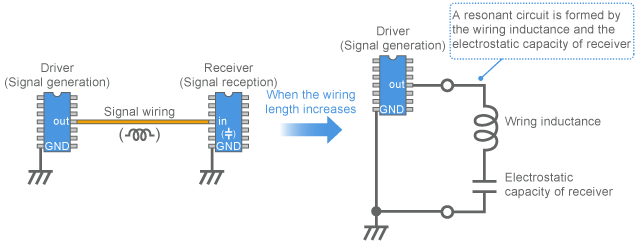Fig. 3-2-8 Resonant circuit model by the wiring of digital signal

##### (3) Power cable and printed board can be factors for resonance

Other than digital signals, various factors that compose the circuit could function as a capacitor or inductor that has not been described in the circuit diagram and cause resonance. So care needs to be taken. Fig. 3-2-9 shows an example.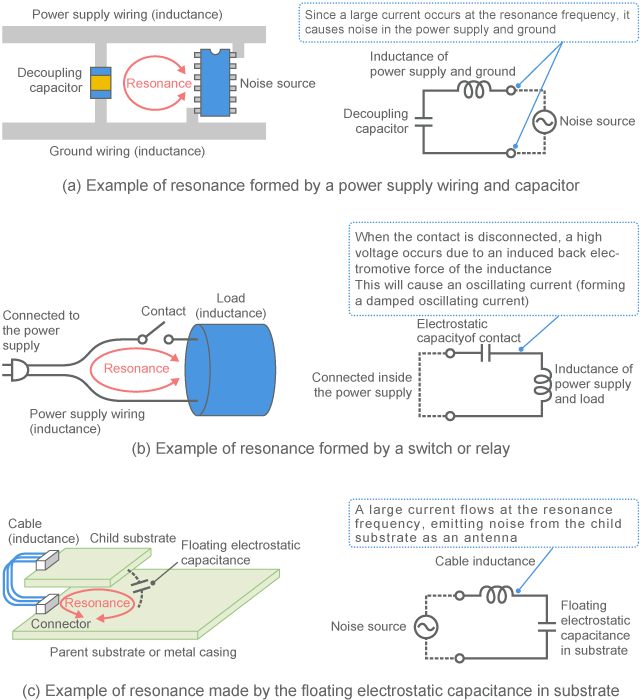Fig. 3-2-9 Example of resonance

#### 3-2-5. Damping by resistor or ferrite bead

##### (1) Damping of series resonant circuit

Resonance can be suppressed by adding a resistor to a resonant circuit. This resistor is called damping resistor. Fig. 3-2-10 shows an example of adding a damping resistor (shown as R in the figure).
When a damping resistor is used in series with the series resonance as shown in Fig. 3-2-10(a), the Q of the resonator is derived as follows  [Reference 1] .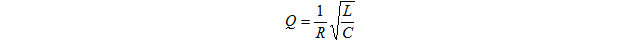(Formula 3-2-2)

For example, we can incorporate the part constants that have been used in the test of Fig. 3-2-4 into this formula. If the signal source output impedance 50 ohms is used for the resistor R , Q = 6.3 is derived, indicating a strong resonance. If the resistor R is higher, Q becomes smaller, thus weakening the resonance. Therefore, you can see that adding a resistor of more than 50 ohms to this point allows weakening the resonance.

Generally in order to suppress the resonance, the resistor is selected so that Q is set to 1 or less.

##### (2) Non-oscillation conditions of series resonant circuit

In order to eliminate overshoot, undershoot or ringing from a pulse waveform such as a digital signal, a resistor that meets the following formula is used to satisfy the the non-oscillation conditions of LCR series resonant circuit.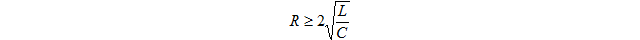(Formula 3-2-3)

Formula (2) leads Q to be 0.5 or less.

##### (3) Damping of parallel resonant circuit

In contrast, when a damping resistor is used in parallel with the parallel resonance as shown in Fig. 3-2-10(b), the Q of the resonator is derived as follows: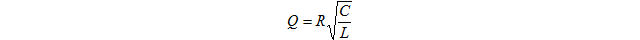(Formula 3-2-4)

In this case, the smaller the resistor, the weaker the resonance becomes.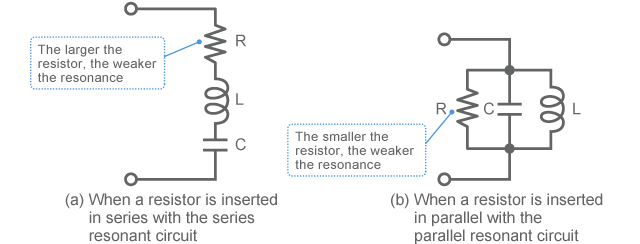Fig. 3-2-10 Example of damping by a resistor

#### 3-2-6. Damping of digital signal

##### (1) Damping resistor and impedance matching resistor

When using a damping resistor to prevent the resonance caused the wiring of the digital circuit shown in Fig. 3-2-8, it is usually used in series with the wiring as shown in Fig. 3-2-11. In so doing, the greater the resistor, the higher the resonance suppression effect becomes. However, if the damping is excessive, it will cause side effects such as signal attenuation and slow rise of pulse waveform. Therefore, the appropriate resistor should be chosen based on the balance between noise suppression and circuit operation. If the wiring can be regarded as a transmission line, this operation can be carried out in a smart manner by using the impedance matching concept described in the next section.

##### (2) Damping by a ferrite bead

As the examples are shown in Section 2.4.7, Fig. 3-2-6, and Fig. 3-2-7, ferrite beads are often used for damping in the EMC measures. In this case, the part should be chosen so that the resistance ( R ) component of the ferrite bead can satisfy Formula (2) at the resonance frequency. Since a ferrite bead has frequency characteristics in impedance, resonance can be suppressed while minimizing the influence on the signal waveform. In addition, it can draw a larger direct current than that a resistor can do.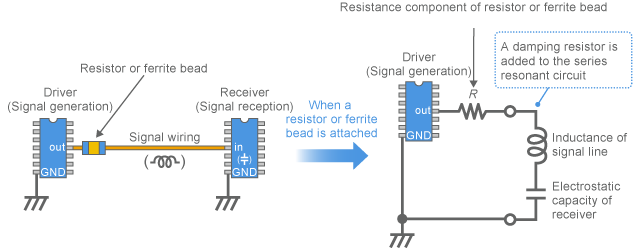Fig. 3-2-11 Damping of digital signal

### “3-2. Resonance and damping” - Key points

• Resonance can be series resonance or parallel resonance
• Series resonance makes the impedance minimum (ideally zero) at the resonance frequency
• Parallel resonance makes the impedance maximum (ideally ) at the resonance frequency
• At the resonance frequency, noise is likely to cause a problem due to its maximum voltage or current
• Damping resistors or ferrite beads are used to suppress resonance